## Introduction

Just starting out with the Raspberry Pi and electronics? Feel like a lot of books explain everything in a difficult way?

In this guide, we'll provide a simple overview and explanation on what they are and what they do, with some theory thrown into the mix. We'll start with a simple blinky LED program, then create a temperature monitor with the DHT11 temperature and humidity sensor.

Once you have finished this first of three guides, you will have a better understanding of just what these components are about, and how to safely and properly use these basic electronic components with the Raspberry Pi.

1. ## Step 1 Overview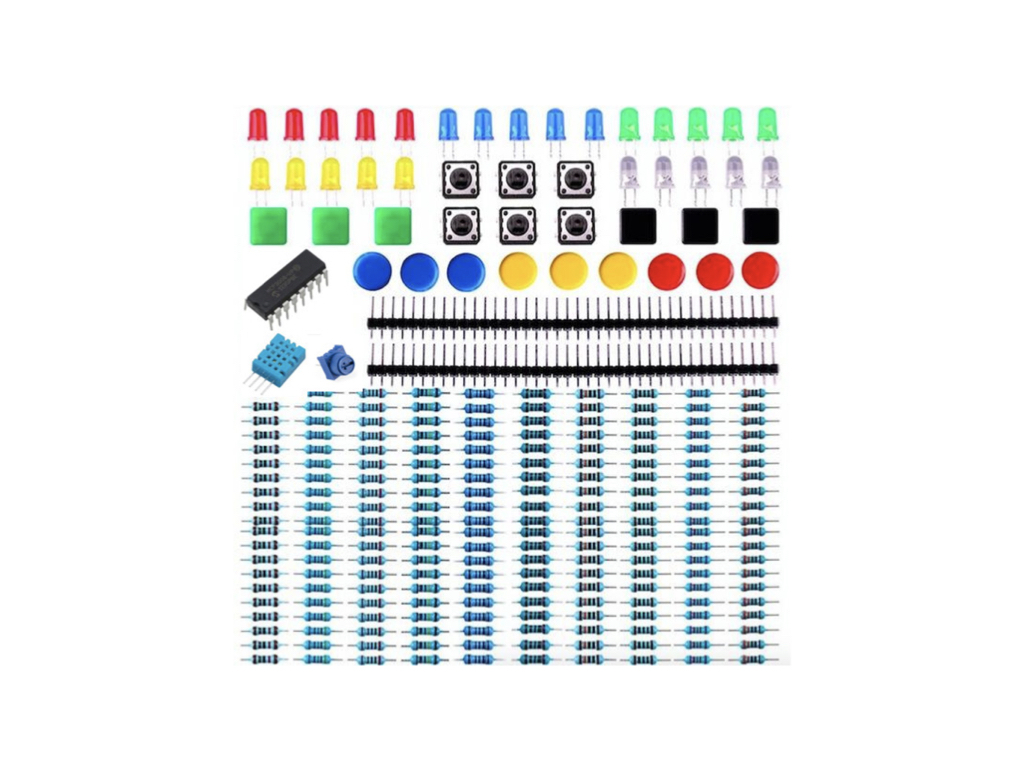• Just starting out with the Raspberry Pi and electronics? Feel like a lot of books explain everything in a difficult way? In this guide, we'll provide a simple overview and explanation on what they are and what they do, with some theory thrown into the mix.

Once you have finished this first of three guides, you will have a better understanding of just what these components are about, and how to safely and properly use these basic electronic components with the Raspberry Pi.

• The STEM Components for Raspberry Pi Kit has everything you'll need to complete the exercises in this guide.

2. ## Step 2 Resistor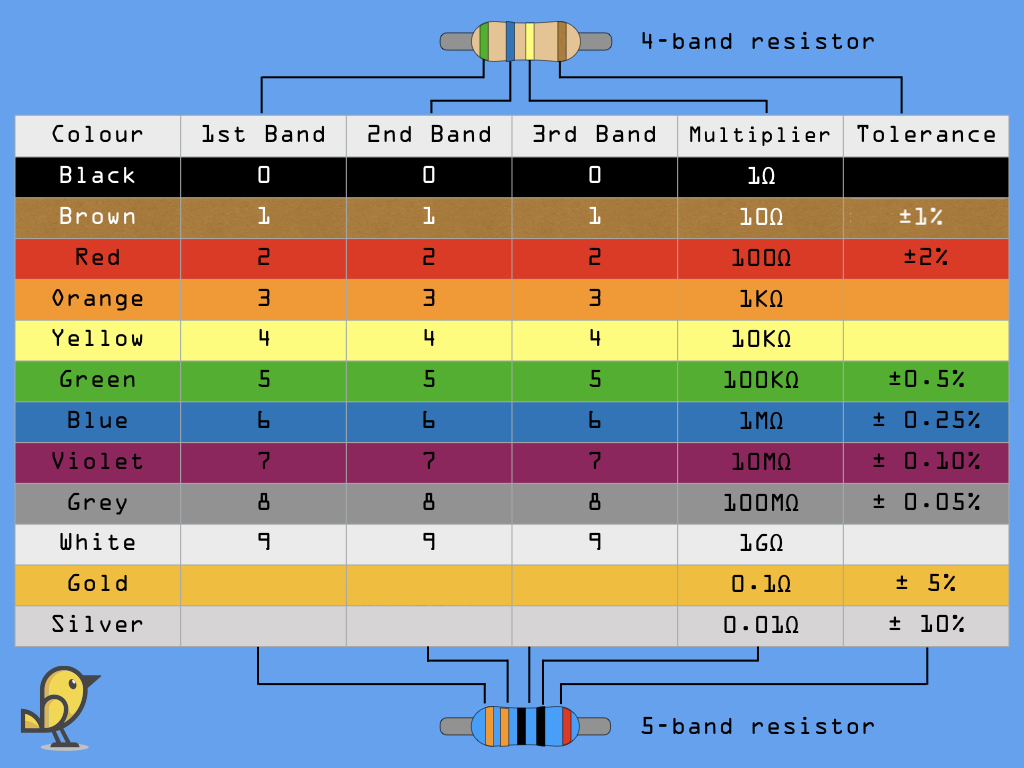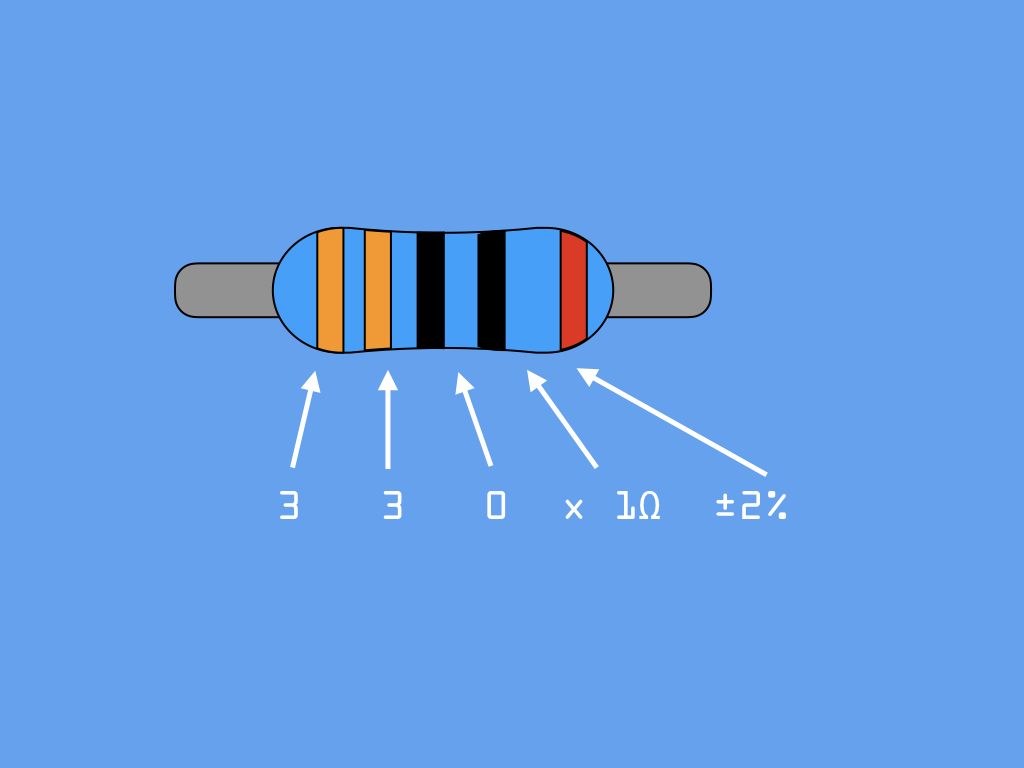• One of the most fundamental components in electronics is the resistor. Resistors can be made of either carbon, metal, or metal-oxide films. Consisting of two wires attached at opposite ends of a relatively poor electrical conductor, one of its main purposes is to limit or control the rate of electron flow.

• As conductivity increases, the resistance decreases. So, conductivity and resistance have an inversely proportionate relationship to each other.

• Resistance is expressed in ohms (Ω). The higher the resistance in ohms, the more opposition it offers to the movement of electrons.

• Take a look at the STEM Components for Raspberry Pi and you'll see resistors that vary in their resistance.

10R stands for ... 10Ω
100R for 100Ω
220R for 200Ω
330R for 330Ω
1K for 1kΩ or 1000Ω
2K for 2kΩ or 2000Ω
5K1 for 5.1kΩ or 5100Ω
100K for 100kΩ  or 100,000Ω
1M stands for 1 Mega Ohm which is 1,000,000Ω

• The coloured stripes represent the values of the resistor. For example, the diagram shows a 330 ohm resistor.

Resistors are not perfect. Please note that the value indicated by the stripes painted on the resistor provides an estimate of the actual resistance. The actual resistance varies by a percentage that depends on the tolerance factor of the resistor.

The blue-coloured body of a resistor is often an indication that its tolerance is 1% or 2%. You may have also seen beige-coloured bodied resistors, these often indicate a tolerance of 5%. Also the blue-bodied resistors contain metal-oxide film elements, while the beige-bodied resistors contain carbon film.

3. ## Step 3 LED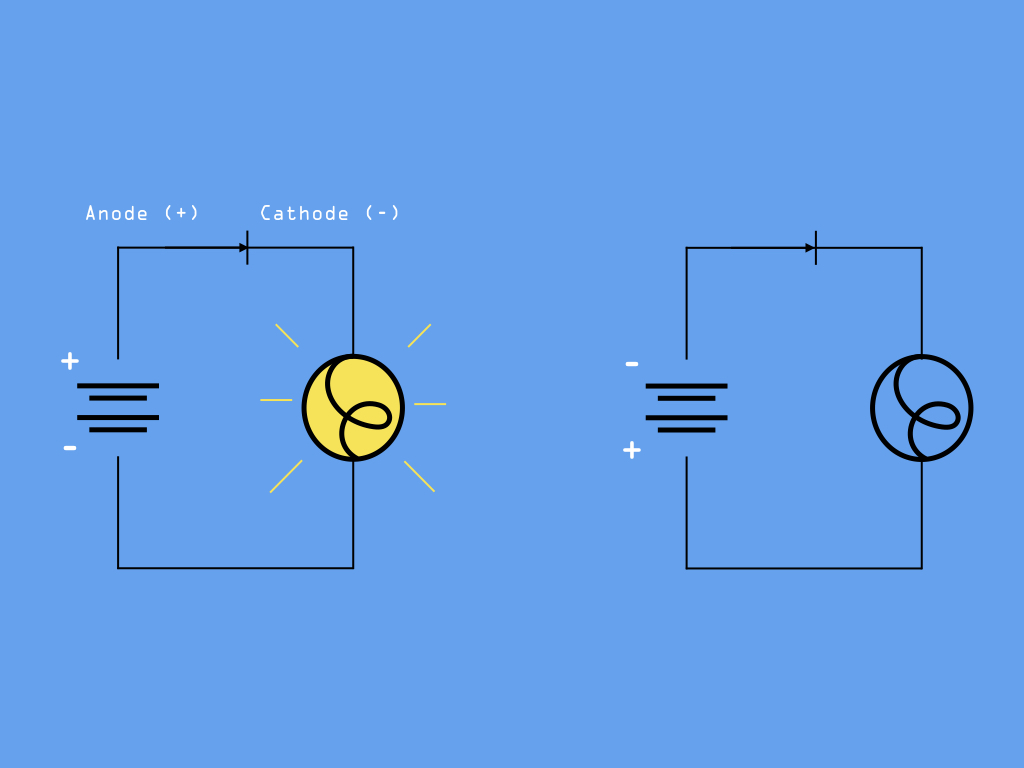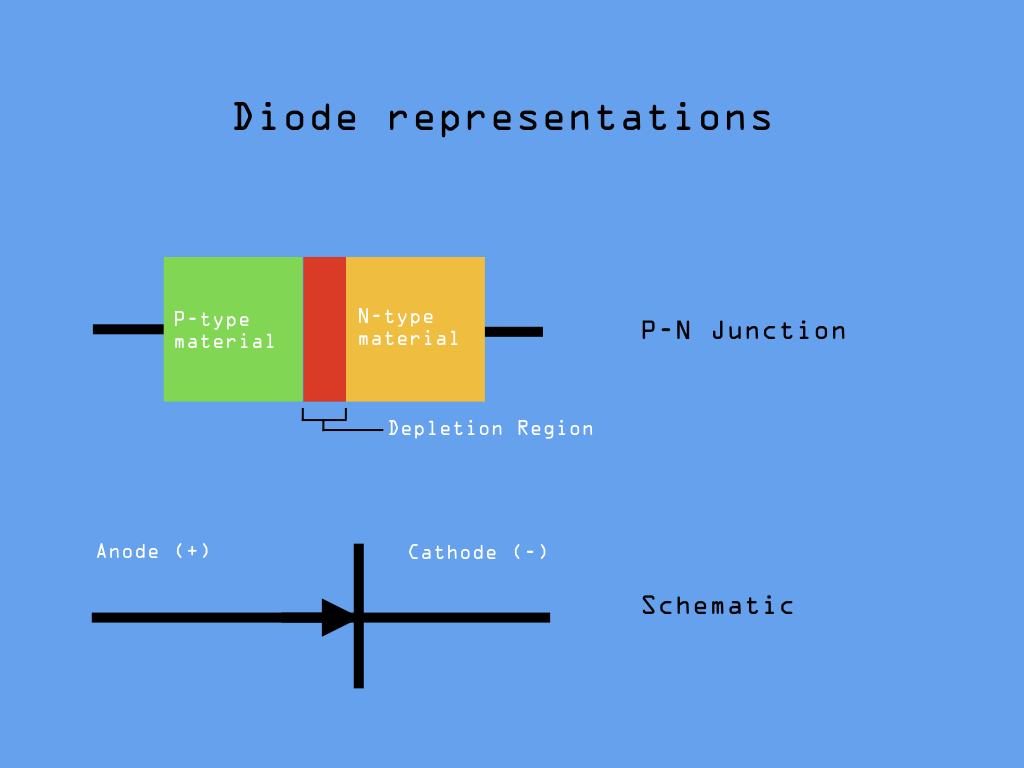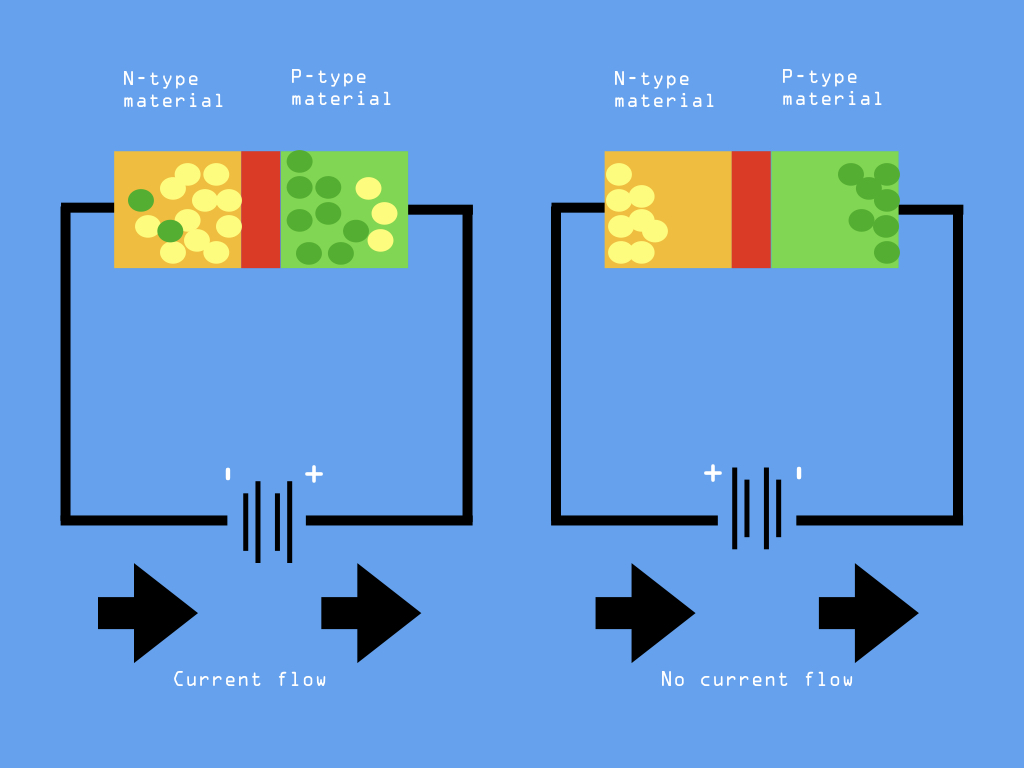• An LED stands for light-emitting diode. Before we talk about what an LED is, let's talk about diodes.

Diodes are one of the simplest but most commonly found semiconductor devices. Semiconductors are materials that conduct current, but only partly. The conductivity of a semiconductor is somewhere between an insulator (conducts no electricity) and a conductor (which has almost full conductivity). Common examples of semiconductors are silicon, germanium, or gallium arsenide. Gallium arsenide is the second most common semiconductor, and can be found used in laser diodes and solar cells.

• The diode is a two-terminal device; it has an anode also known as the positive terminal, and a cathode which is its negative terminal. Take a look at the STEM components for Raspberry Pi and you will find red, blue, green, yellow and white LEDs. One end or leg of the LED will be longer than the other, this longer end is the anode.

• A diode is comprised of a P-type semiconductor and an N-type semiconductor. Between the two is a region that acts as a barrier to electric current.

Add a battery to our circuit as shown, and the voltage from a battery forces charges in the N and P layers towards the junction region between them. Current then begins to flow! Yes, by themselves, the P-type and N-type materials aren't very interesting but if we bring them together, a useful thing happens: current can flow through the resulting semiconductor, but only in one direction.

This brings us to our next point about diodes: they are unidirectional so they only allow current to flow through them in one direction, known as the forward direction. This happens when the anode of the diode has a higher positive potential than the cathode.

If we reversed the polarity (by for example, flipping the battery in the circuit as shown in the diagram) then current flow is blocked. The diode is now reverse biased. Here, the N and P layers are attracted away from the central junction, which becomes a depletion region, unable to pass significant current.

• In other words, a diode may be thought of as like a switch: it is “closed” when forward-biased and “open” when reverse-biased.

• Please note that even though we mentioned no current flowing when reverse-biased, this is only theoretically the case. We've talked about tolerance in resistors, likewise a diode is not 100% efficient.

When it is forward biased and current is flowing, it imposes a voltage drop of 0.7V for a sillicon-based diode. On the other hand, when reversed biased, a small amount of current manages to get through. This is known as leakage and is almost always less than 1 mA.

• While there are many different types of diodes, we will focus on the light-emitting diode for now. There are LEDs from single-coloured, multi-colour, low-power, and high-powered ones. The LEDs from our STEM components for Raspberry Pi are single-coloured LEDs that can be used for indication or illumination, as visual feedback from our circuit.

• While it is simple to light an LED, it needs to be done properly in order to protect the components in your circuit. For example, when connecting it to the Raspberry Pi, this needs to be done with a resistor to prevent it from drawing too much current and burning itself out.

Different LEDs will be rated at a certain amount of current. Your Raspberry Pi supplies another amount of current and may even try to supply far more than it should. Thus, your resistor choice should be appropriate for these values. How to choose a resistor for your circuit? You can calculate this with Ohm's Law

4. ## Step 4 Ohm's Law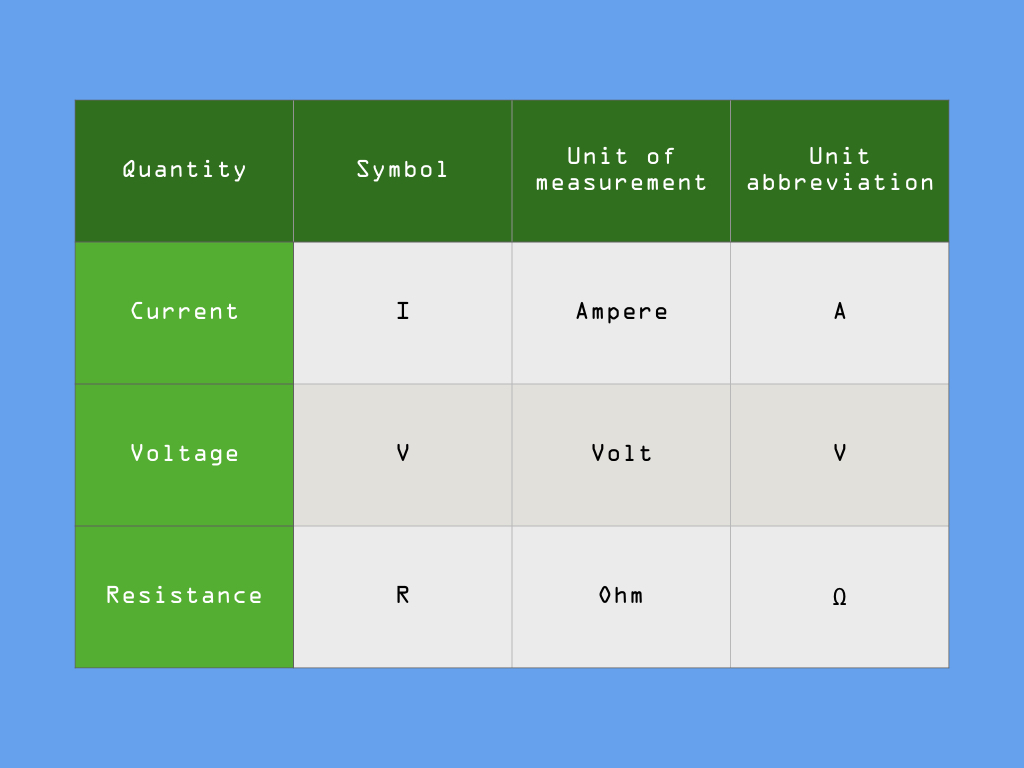• To understand how to calculate the resistor value for our circuit, we'll first consider Ohm's Law, Discovered by Georg Simon Ohm and published in his 1827 paper, The Galvanic Circuit Investigated Mathematically.

• According to Ohm's Law, the voltage across a resistor is directly proportional to the current flowing through it.

V = iR

Voltage is measured in Volts (V)
Current is measured in Amperes (A)
Resistance is measured in Ohms (Ω)

We'll be using this equation a lot when working with circuits.

• So how do we find the voltage or current values for our circuit, or rather a better starting question to ask would be, "How much current does this LED require?"

To find out, we'll need to take a look at the forward current of the LED, which tells us how much current is required to flow across the LED's leads, from anode to cathode, in order for there to be sufficient current to light up the LED.

We also need to look at the forward voltage of the LED, this is the amount of voltage needed to get current to flow across the LED. Take a look at the 3mm red LED in the STEM Components for Raspberry Pi. This is rated at a max forward current of 20 mA and typical forward voltage of 2.0V.

• Next, we'll need to figure out how much current is supplied by the Raspberry Pi to the LED.

The Raspberry Pi is powered by a 5V power supply, but the processor is actually powered by 3.3V through a voltage regulator. So, the supplied voltage from the Pi is 3.3V.

The LED Forward Current is 20 mA. The LED Forward Voltage for the red LED is 1.8V.

To find the resistor value, we simply take 3.3V - 2.0V then divide it by the max forward current of the LED, which is 20 mA.

Finally, we need to divide this value by 1000 in order to convert milliamps (mA) into amperes (A). The resistance value required is calculated to be 65 ohms.

• Since we do not have a 65 ohm resistor, we'll use the next closest value in our component kit - the 100 ohm resistor.  However, it is recommended to use a higher value just to be safe due to the resistor tolerance. With metal-film oxide resistors, there is a 1 or 2 % tolerance, this means the value can be 1-2% higher or lower than specified. So a 100 ohm resistor could be 98 ohms or 102 ohms. Therefore, it is good practise to pick a higher valued resistor!

• To play it very very safe, we'll use a 330 ohm resistor with the red LED and Raspberry Pi!

5. ## Step 5 Connect the Raspberry Pi to a resistor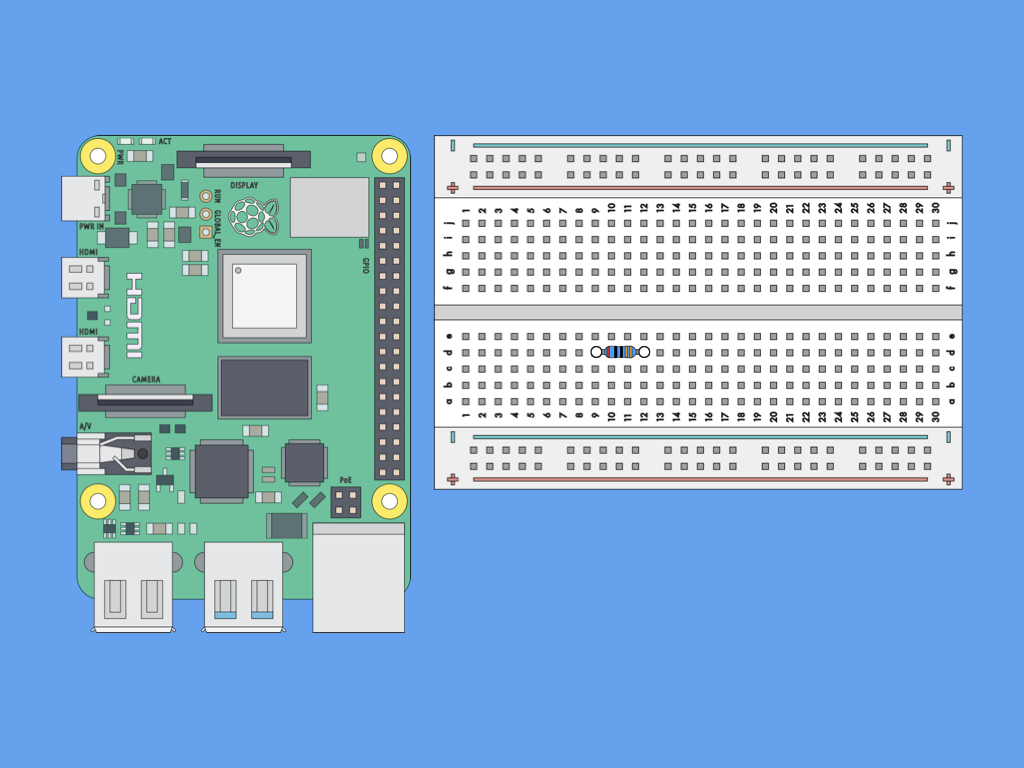• An electric circuit is formed when a conductive path is created to allow charge to continuously move. To create this circuit, let's first attach the 330Ω resistor to the 400-point breadboard as shown.

6. ## Step 6 Connect the Raspberry Pi to an LED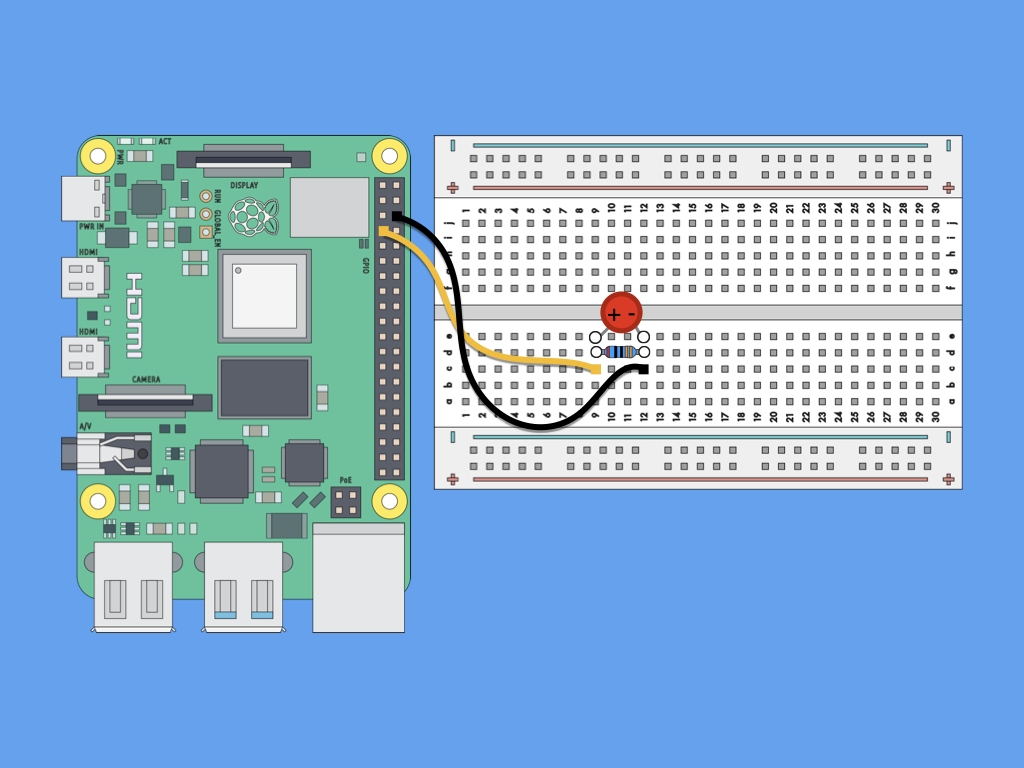• Next, insert the LED into the breadboard.

• Connect a jumper wire from the anode of the LED to GPIO4 of the Raspberry Pi.

• Finally, connect a black jumper wire from the cathode of the LED to GND of the Raspberry Pi.

7. ## Step 7 Basic blinky code

``````from gpiozero import LED
from time import sleep
led = LED(4)
while True:
led.on()
sleep(1)
led.off()
sleep(1)``````
• Create a new python file using the terminal with the command: sudo nano blinky.py

• Copy and paste the following code.

• To save, press CTRL+O and then enter Y.

• Check out our other guides for more information on how to edit text files or using the command line with the Raspberry Pi.

8. ## Step 8 Transistor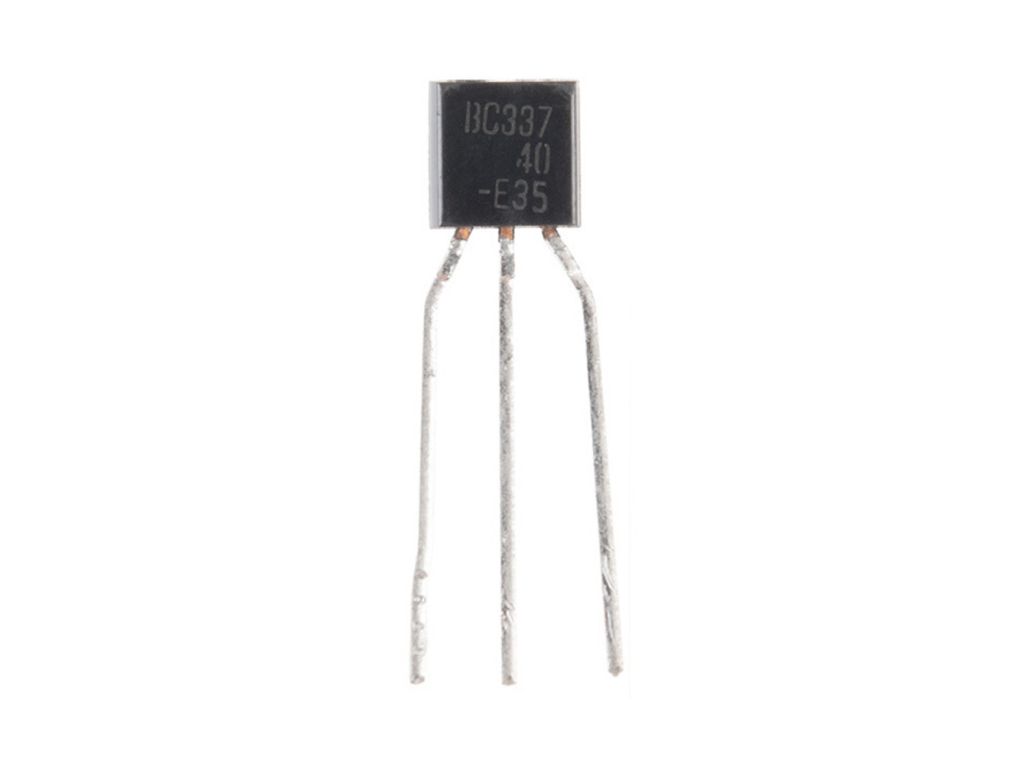• A transistor can be thought of as an electronic switch, as it can turn a current on and off. That's not all, it can also be thought of as a "current regulator".

The transistor lets you control the flow of current; This is done by varying the intensity of a smaller current in one channel, which then changes the intensity of a larger current on a second channel.

You can think of it as an electronic lever of sorts. A lever lets us lift a larger load by exerting a smaller effort. Likewise, that's what a transistor can do: It allows a small current to control a much larger current. After all, it is transistors which replaced vacuum tubes that were formerly used in the amplification of audio signals.

• The type of transistor we'll look a closer look at is one that is commonly used in hobbyist/maker projects, the bipolar transistor. As its name suggests, it has two different types of junctions, p and n. These are just junctions made of two different types of semiconductors, one that has a positive charge (p-type) and the other with a negative charge (n-type). When inspecting the diode before, we mentioned that it had a p-n junction with leads attached to both ends. It is this p-n junction which allows current to flow in one direction, and one direction only.

For the bipolar transistor, it has either NPN or a PNP junctions, that is to say it has three regions instead of two. Due to its interface between each of the regions that forms a p-n junction, you can think of a transistor as a semiconductor with two p-n junctions.

• Take a closer look at the transistor and you'll notice three leads or legs, the collector, the base, and the emitter.

Here's how they relate to the NPN or PNP transistor:

NPN: When a voltage is applied to the base leg of the transistor it allows current to flow from the collector to the emitter. This means you can control a higher current path using logic level signals from a device like a micro-controller.

PNP: When a voltage is applied to the base leg of the transistor it prevents current from flowing from the collector to the emitter.

9. ## Step 9 Integrated Circuit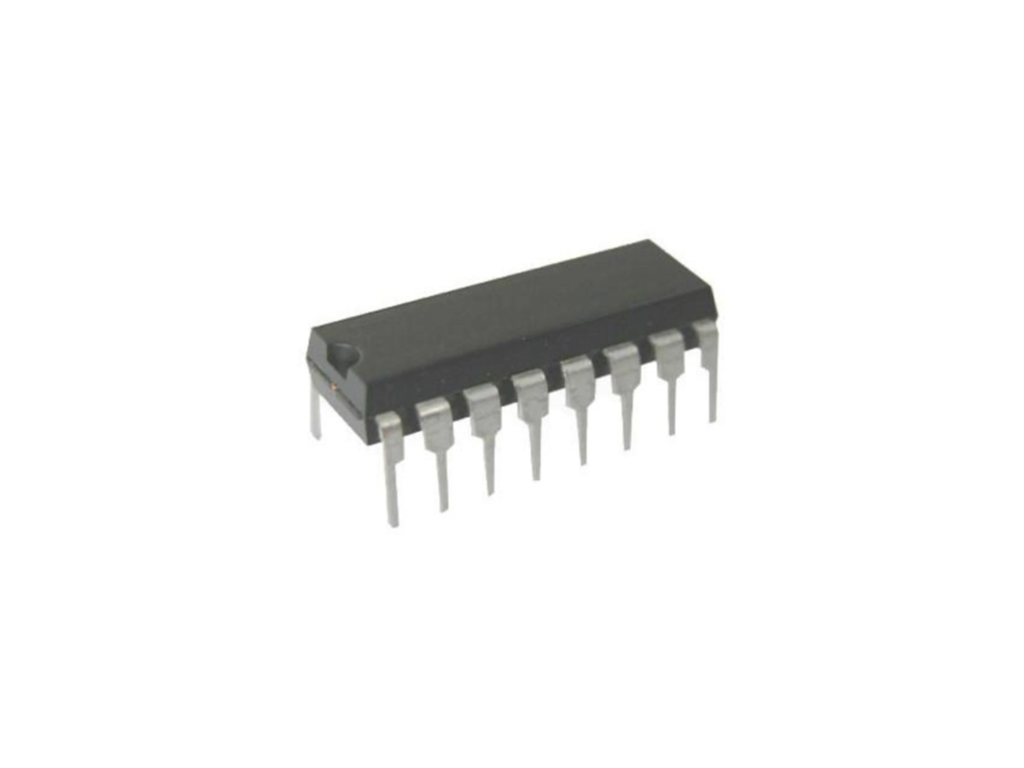• Now that we've talked about transistors, let's talk about integrated circuits. A modern integrated circuit, or chip as some people like to call them, may have over a billion transistors on it.

Besides transistors, integrated circuits consist of many basic electronic components packed together to achieve a common goal. You can think of it as an electronic circuit shrunk to fit inside a chip.

So what does it do exactly? It could be an analog to digital converter such as the MCP3008 found in the STEM Components for Raspberry Pi Kit. Working with components such as light dependent resistors, useful analogue sensors requires the use of an ADC such as the MCP3008.

• To find out how, follow our guide on how to use analogue sensors with Raspberry Pi with a light-dependent resistor.

• Other IC are USB to serial converters, amplifiers, motor controllers and the list goes on.

Another example is the DHT11 temperature and humidity sensor found in the kit which has also got a little IC in it.

To figure out what a particular IC does exactly, read its datasheet!

10. ## Step 10 Connect resistor to Pin 1 and 2 of DHT11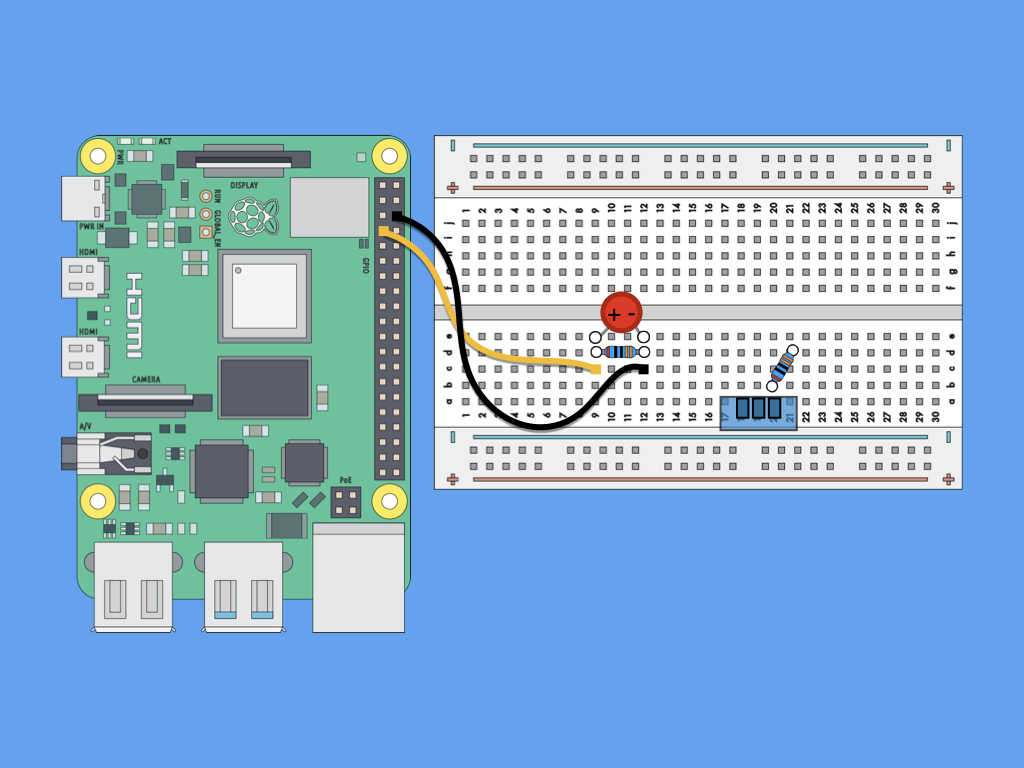• With all this talk about integrated circuits, let's put one to use right now. While the Raspberry Pi doesn't have a temperature sensor, it does have access to the internet. So let's build a temperature monitor with the DHT11 temperature and humidity sensor.

First, insert the DHT11 into the breadboard as shown.

• Next, bridge DHT11 Pin 1 and Pin 2 with a 10k Resistor.

11. ## Step 11 Connect Pin 1 of DHT11 to 5V on Raspberry Pi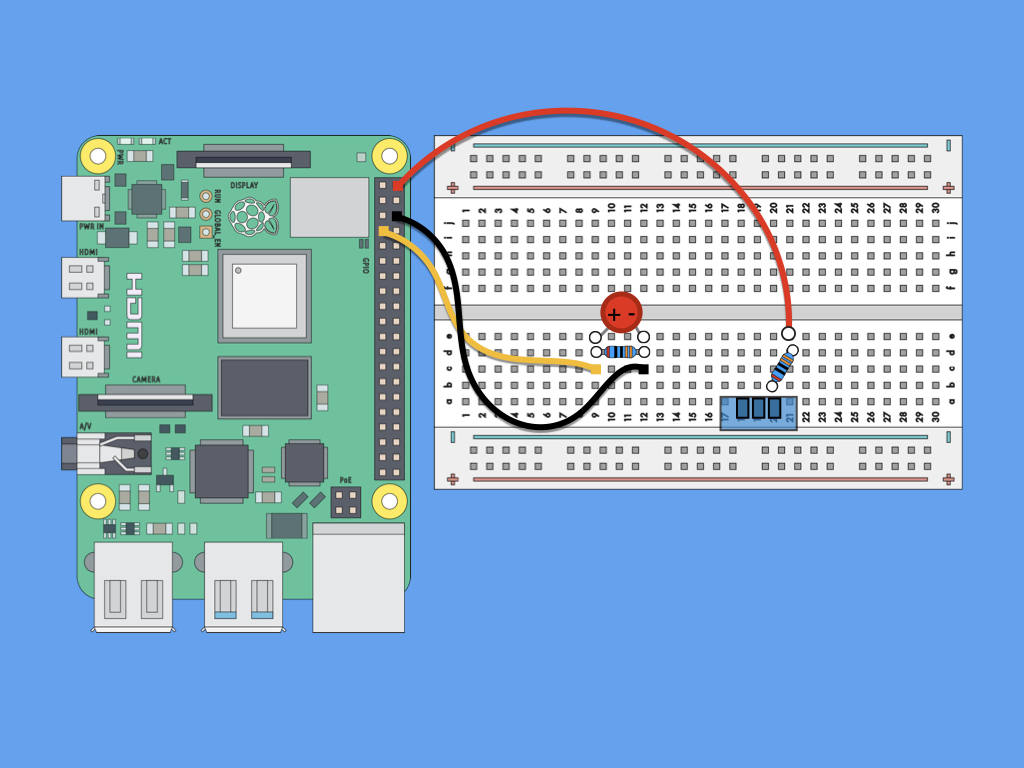• Connect 5V to Pin 1 of DHT11.

12. ## Step 12 Connect Pin 2 of DHT11 to GPIO17 of Raspberry Pi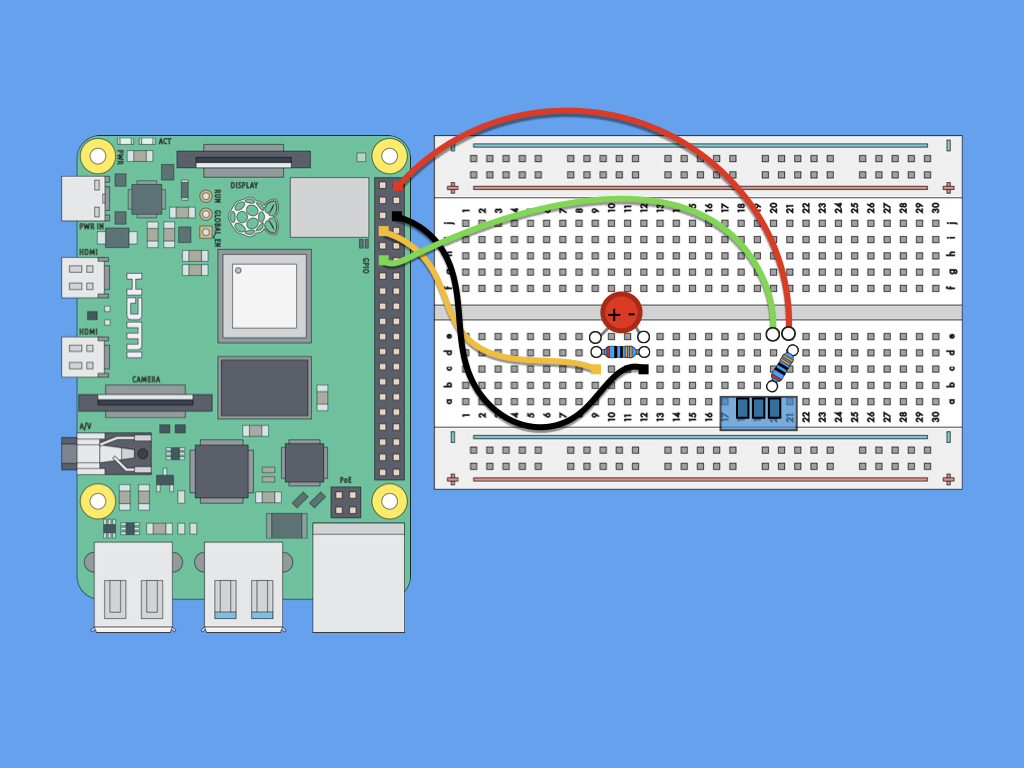• Finally, attach a jumper wire from Pin 2 of DHT11 to GPIO17 on Raspberry Pi.

13. ## Step 13 Connect Pin 4 of DHT11 to GND of Raspberry Pi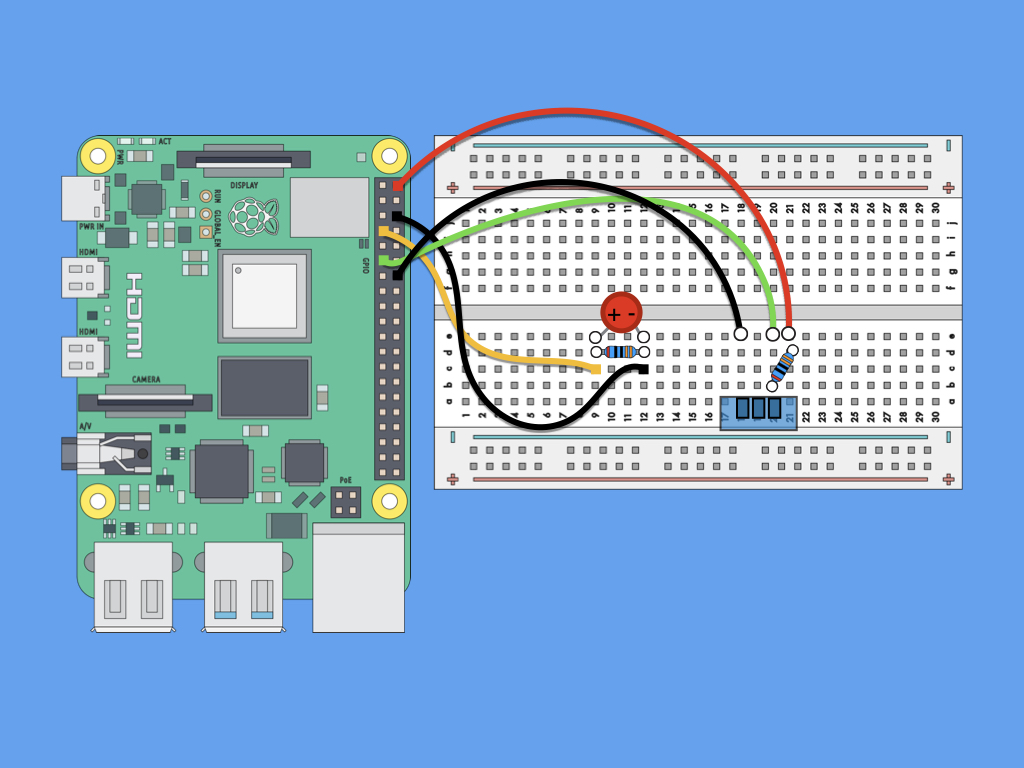• Next, attach a jumper wire from Pin 4 of DHT11 to another GND pin of Raspberry Pi.

14. ## Step 14 Install git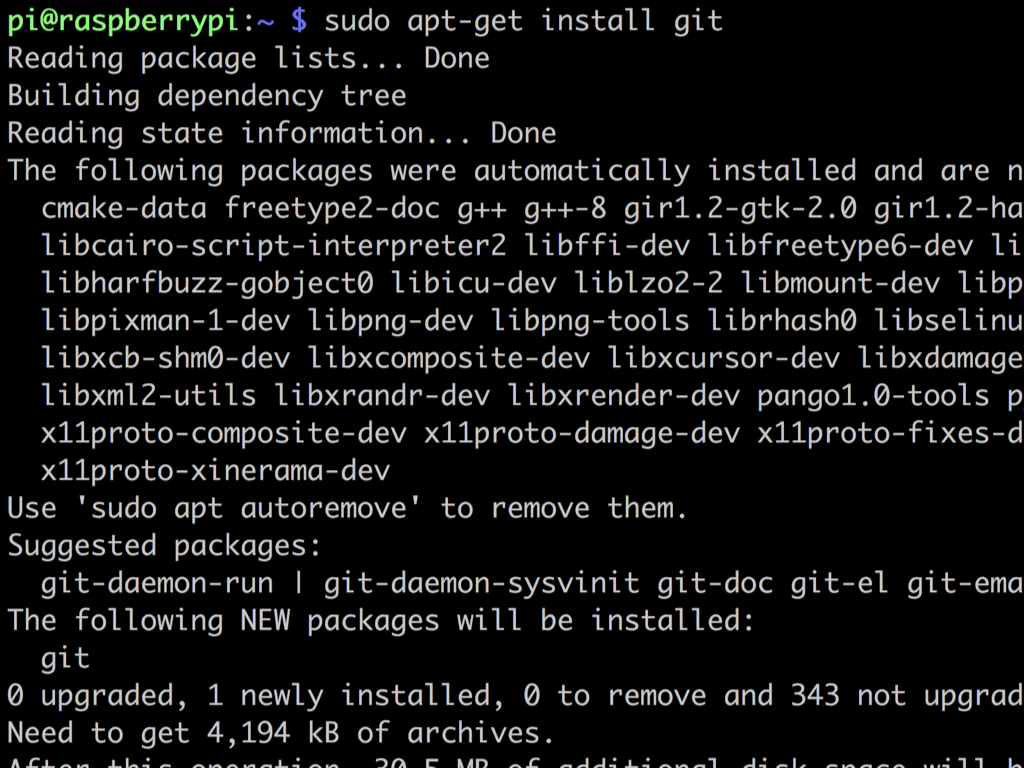• First, open up a new terminal window and enter the following command to install git: sudo apt-get install git

• If hit with an error message, run the command sudo apt-get update first.

15. ## Step 15 Copy DHT11 Python Library repository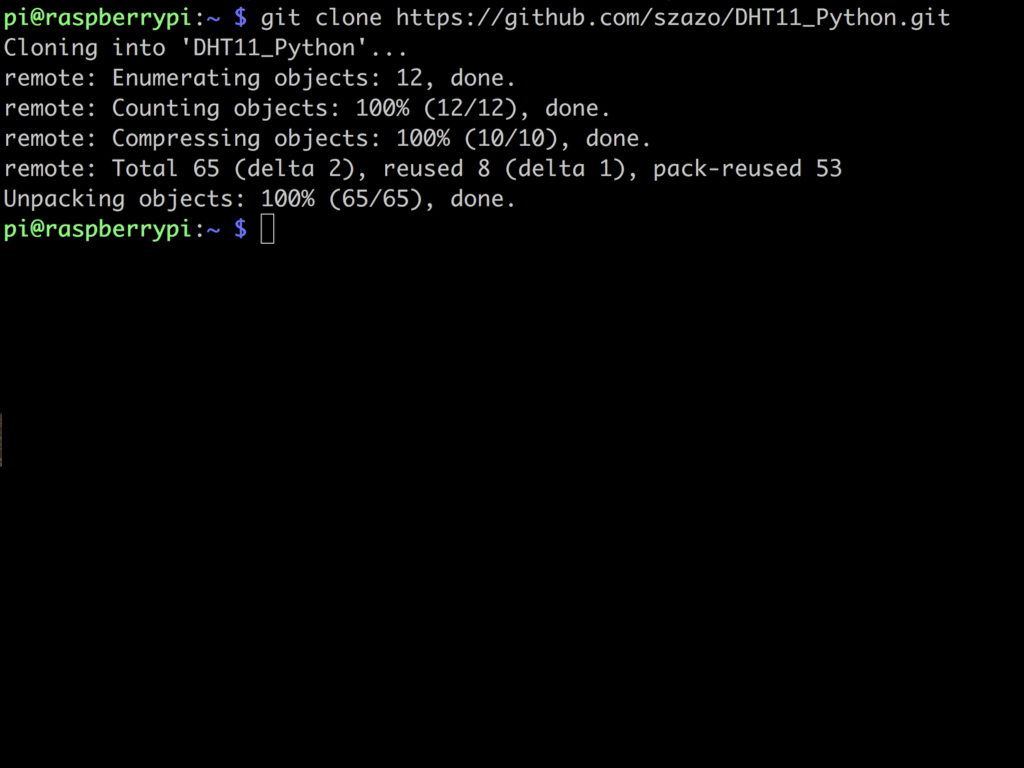16. ## Step 16 Navigate to folder DHT11_Python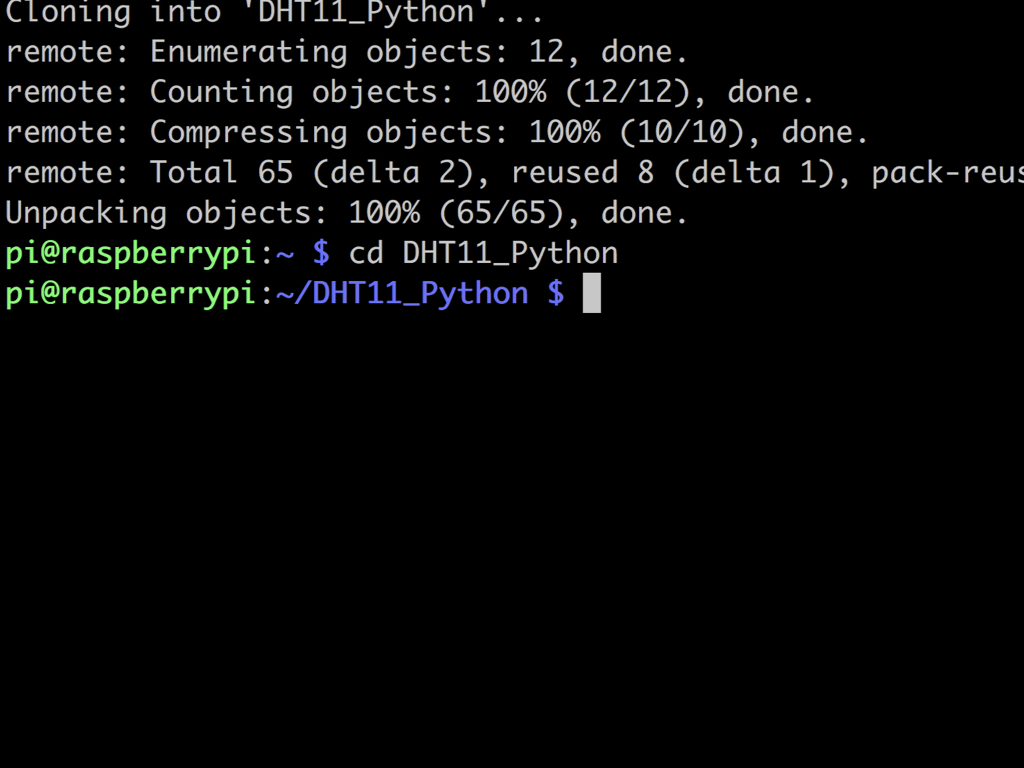• Next, enter the command: cd DHT11_Python

17. ## Step 17 Edit example code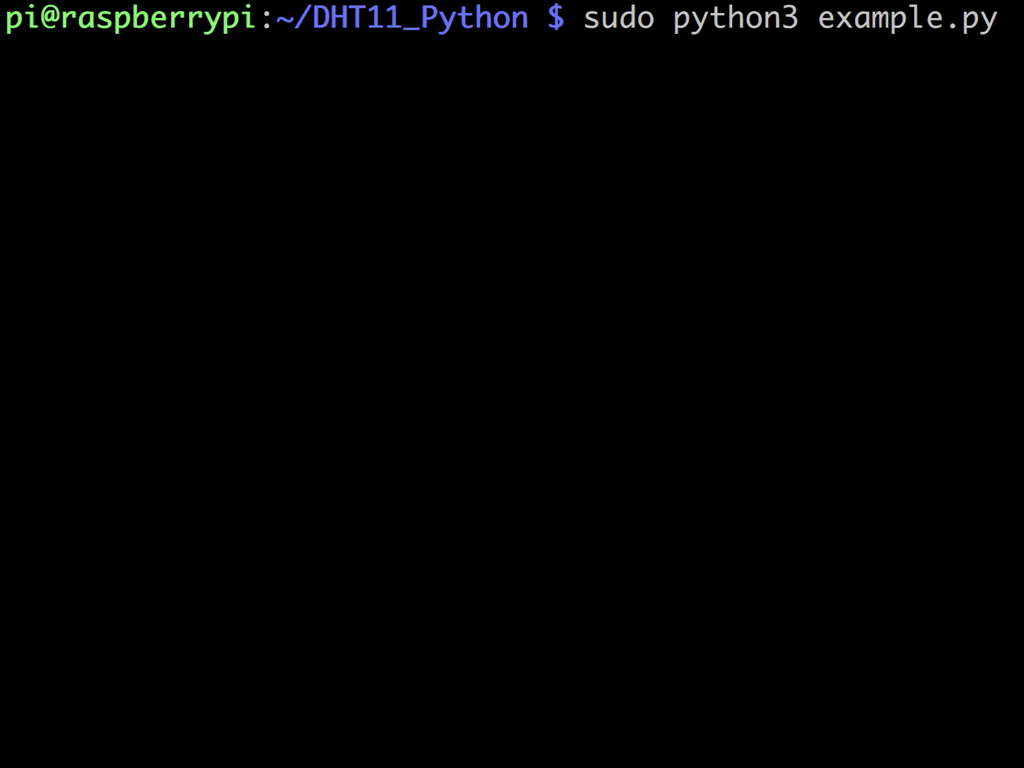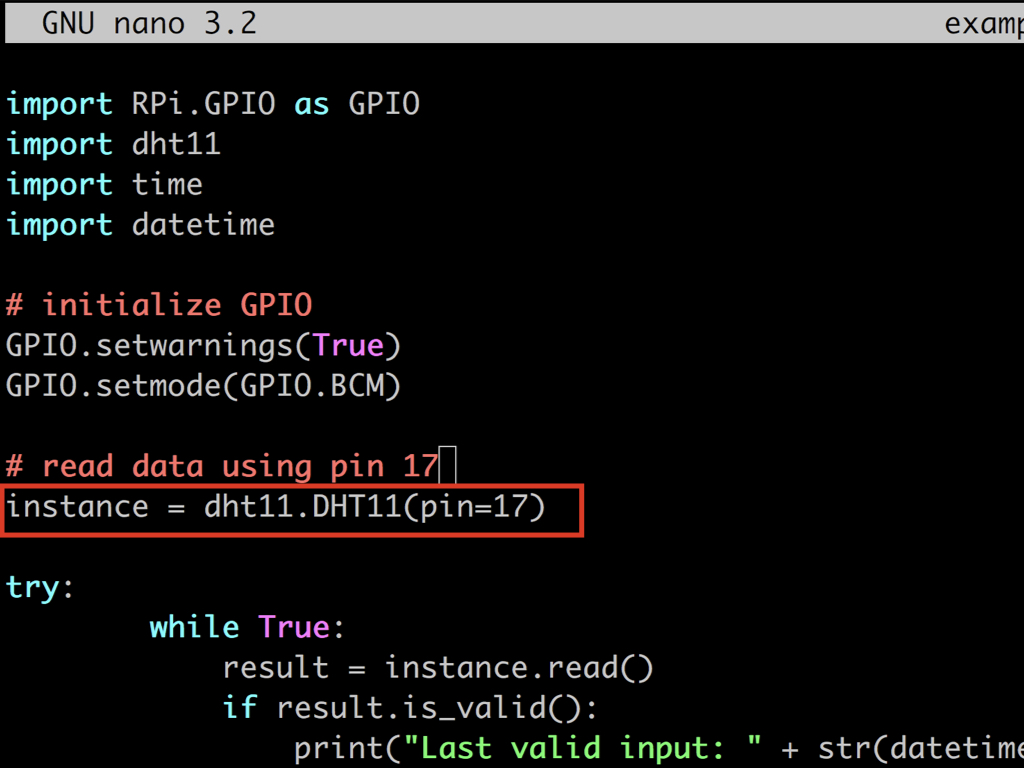• sudo nano example.py

• Since the DATA pin (Pin 2) of the DHT11 sensor was connected to GPIO17, we'll need to edit instance = dht11.DHT11(pin=14) and change it to "pin=17"

• Save the code, and run it with sudo python3 example.py

18. ## Step 18 Conclusion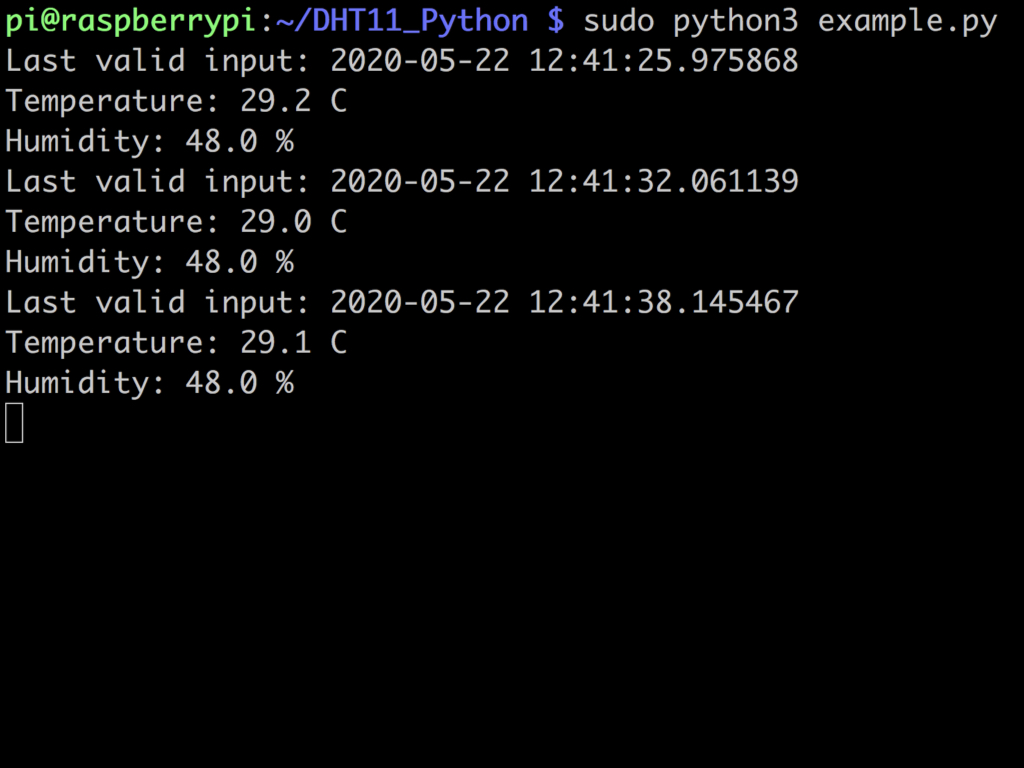• In this guide, we've briefly talked about resistors, ohm's law, diodes specifically the light-emitting diode (LED), bipolar transistors, and integrated circuits.

As briefly mentioned in the section on integrated circuits (IC), the MCP3008 is an analog-to-digital converter IC. As the Raspberry Pi is not able to read electrical analog data, one way to get around this is to use the MCP3008. A second way is through the use of a simple charging-discharging circuit via a capacitor. The charging-discharging circuit will allow continuous data to be read by the Raspberry Pi through what is called a step response technique.

In the next guide, we'll show you how to use the MCP3008 with the potentiometer found in the STEM components for Raspberry Pi kit, to convert analog signals into digital signals and create a light show. We'll also show you how to use a capacitor for analog to digital conversion.